# Round a Number in Microsoft Excel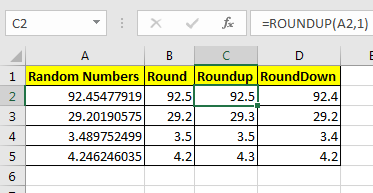So here we are, with calculations that give long decimal results.The value of PI is 3.1415926. But most of our work is done by just 3.14. This is called rounding. Let’s see how we can round the numbers in excel.

Let's say in cell A2 we have 92.45477919. To limit these decimal points you can go to Home Tab? Decrease Decimal and click it until you reach your desired number of decimals.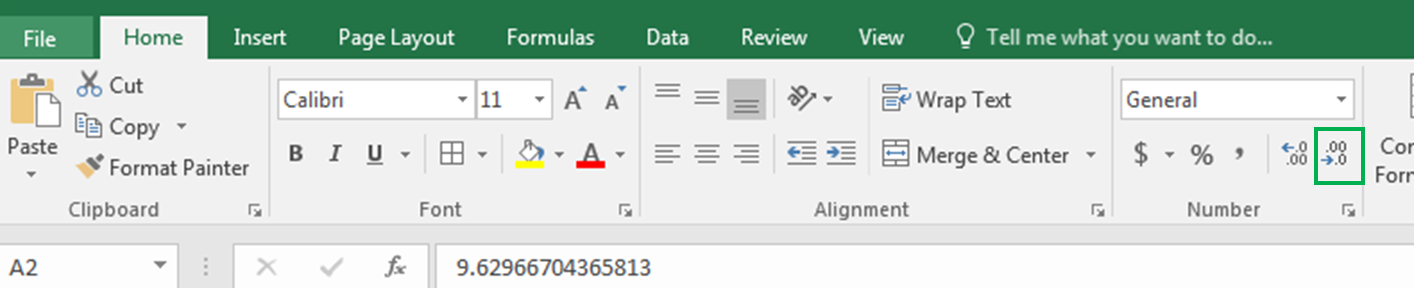Wouldn’t it be easy and time-saving if you can do it with a formula?

Excel provides three formulas for Rounding Numeric numbers:

 ROUND (value, num of decimal digits) ROUNDUP(value, num of decimal digits) ROUNDDOWN (value, num of decimal digits)

Examples will make it easy.

#### ROUND Function in Excel

The round function simply limits decimal value. It takes two arguments. First the value (the number that needs to be rounded) and second the number of decimals.

For example here in Cell A2, I have a random decimal number generated by RAND() function and then multiplied by 100. Now in Cell B2 I want to have only 1 decimal digit, so I write:

 =ROUND(A2,1)

You can see the result in A2 in the below image.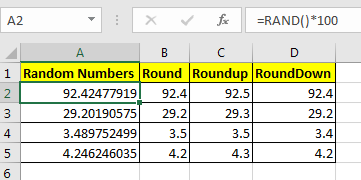It shows only one digit.

 Pro Note: if the second decimal digit was greater than or equal to 5 than ROUND function would increase the first decimal by 1. You can see it in Cell B4 in the above image.

#### ROUNDUP Function in Excel

Same as the ROUND function of Excel, the ROUNDUP function limits the decimal points shown but it always increases the last decimal digit by one.

This, too, takes two arguments. First the value (the number that needs to be rounded) and second the number of decimals.

Now in Cell C2, I want to have only 1 decimal digit but I want to increase it by one to compensate the leftover digits. So I write this formula in C2:

 =ROUNDUP(A2,1)You can see that the value is rounded (92.5) to one decimal and it is increased by one.

#### ROUNDDOWN Function in Excel

The ROUNDDOWN function just shows the last decimal digit as it is and makes all following decimal digits 0. In short, it prevents the rounding up of a number.

In Cell D2 I have written this formula:

 =ROUNDOWN(A2,1)

You can see that 92.454779 is now 92.4. Similarly, all numbers in column D are shown as they depend on defined decimal digits.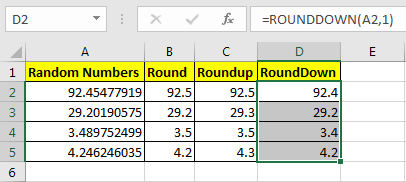Pro Note: This does not decrease the last digit.

Now you know how to harness control of these decimal digits to your desired number digits. I tried to explain ROUND functions in Excel in the most precise way. These Rounding functions are available in Excel 2016, 2013, 2010 and in older Excel versions.

Let me know if that would be helpful. If your problem is not solved then post it in the comments section.

Scenario:

We like to work on well maintained data. So cleaning and brushing data is the most important part before working with data. Generally, we are stuck at time fields and not able to understand how to round up or round down time values.

How to solve the problem?

To round down time values we use MROUND function. Excel stores time values as numbers. In order to handle these values, we go the basic mathematical formulation as shown below.

Generic formula:

 =MROUND(time,"round_to")

time : time value to round

round_to : round to time value

Example :

All of these might be confusing to understand. Let's check the formula via running some examples. Here we have a some random time values and we need to round the time values as per given argument. Here we use the formula to round down to nearest 15 minutes.

Use the formula with explanation

 =MROUND(C3,"0:15")

Explanation

1. =MROUND(C3,"0:15"), function operates the "0.15" value as 15 / (60 *24).
2. The function rounds off the time value to 15 / (60 *24).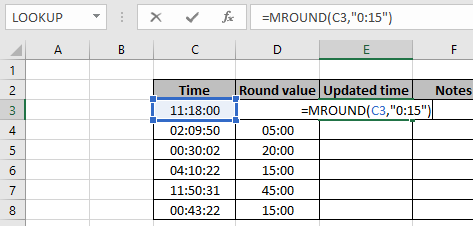Here the time values are provided using cell reference and value to round to be given with quotation marks ("). Press Enter to get the time value.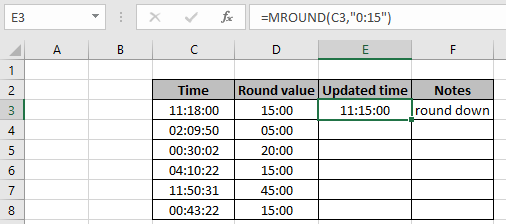As you can see the time value is round down to nearest .15 value. Now try the formula with different time values and round values. Make sure to use the time format carefully.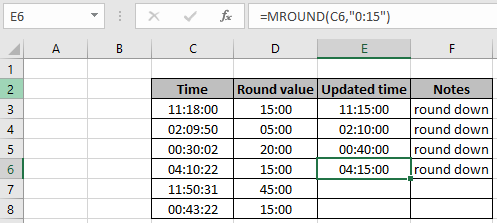As you can see, these values are rounded down to the mentioned round values. If you wish to round up the time function. Use the CEILING function, to round down the time value.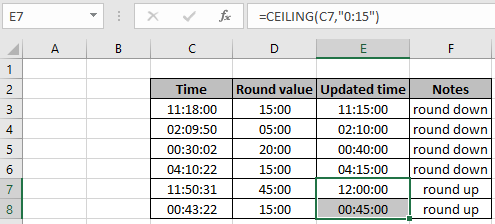As you can see, this how you can round up or round down time values using the excel functions.

Mathematical formulas

Retail price = Price / ( 1 + percent )

Sales Tax = retail price * percent = Price - Retail price

ROUND function with financial formulas

ROUND function rounds off the number extracted by formula to the given decimal points.

ROUND Function syntax:

 =ROUND ( price / ( 1 + percent ), 2) ####Retail price
 = ROUND ( percent * retail , 2 ) ####Sales tax price

Example :

All of these might be confusing to understand. Let's understand how to use the function using an example. Here we will run the formula on some samples. The tax is given = 7.75%

Use the formula:

 =ROUND(A2/(1+7.75%),2)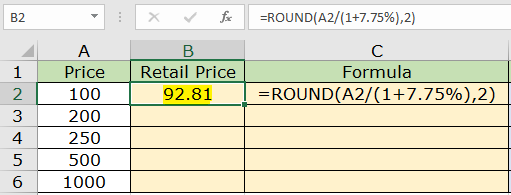The retail price for the 100\$ price comes to be 92.81\$ . Now copy the formula to the other cells using the CTRL + D to the required cell.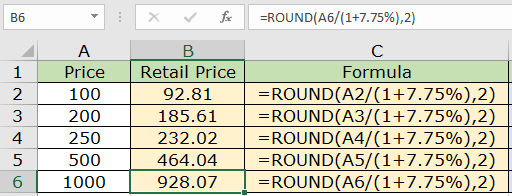As you can see, we got all the retail prices for the total price.

Now we can get the Sales Tax price using the Retail price using the below stated formula.

Use the formula:

 =ROUND(A2/(1+7.75%),2)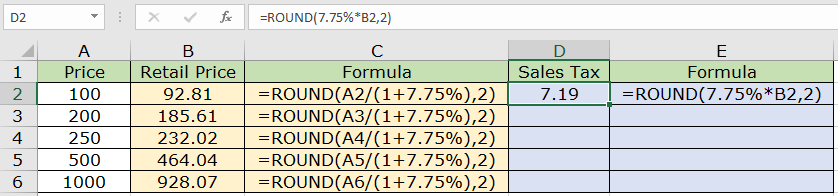Now we see the 7.19\$ tax on the 100\$ price (92.81 \$ retail) amount for a product. Now copy the formula to the other cells using the Ctrl + D shortcut key.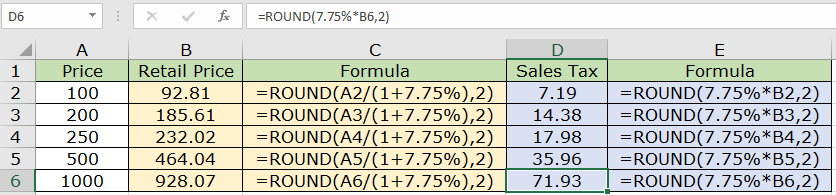As you can see all the detail prices for the given product price with the formula in the adjacent cell. You can use the ROUNDUP, ROUNDDOWN, CEILING, FLOOR function to round off the number to the required side of the number

Here are all the observational notes using the Round function in Excel
Notes :

1. The formula only works with numbers.
2. The formula returns the nearest last multiple given input any decimal number.
3. Negative significance is only allowed when number itself is negative else the formula returns #NUM! error.
4. Blank cell or 0 as significance value returns #DIV/0! error.
5. The function returns #VALUE! Error if the date or time value is invalid.
6. Excel stores time value as numbers. So check the format of cell while operating this formula.
7. Careful while Input time values 04:10:22 means 4 hours, 10 minutes and 22 seconds.

Hope this article about Round a Number in Microsoft Excel is explanatory. Find more articles on date and time formula here. If you liked our blogs, share it with your fristarts on Facebook. And also you can follow us on Twitter and Facebook. We would love to hear from you, do let us know how we can improve, complement or innovate our work and make it better for you. Write to us at info@exceltip.com.

Related Articles

How to use the FLOOR function in Excel | rounds down the number to the nearest specified multiple using the FLOOR function in Excel

How to use the ROUND function in Excel | Rounds off the given number to the nearest num_digit decimal using the ROUND function in Excel

How to use the ROUNDUP function in Excel | Rounds up the given number to the nearest num_digit decimal using the ROUNDUP function in Excel

How to use the ROUNDDOWN function in Excel | Rounds down the given number to the nearest num_digit decimal using the ROUNDDOWN function in Excel

How to use the MROUND function in Excel | rounds off the number to the nearest specified multiple using the MROUND function in Excel.

Popular Articles :

How to use the IF Function in Excel : The IF statement in Excel checks the condition and returns a specific value if the condition is TRUE or returns another specific value if FALSE.

How to use the VLOOKUP Function in Excel : This is one of the most used and popular functions of excel that is used to lookup value from different ranges and sheets.

How to use the SUMIF Function in Excel : This is another dashboard essential function. This helps you sum up values on specific conditions.

How to use the COUNTIF Function in Excel : Count values with conditions using this amazing function. You don't need to filter your data to count specific values. Countif function is essential to prepare your dashboard.

1.Thirpal Das Khatri

Very useful for beginners

2.Hi Sophannasoth,

Assume you have numbers in cell A1 and A2, use the below function in cell B1 and paste the same in cell B2 you will get required result by using this formula...
=Floor(A1+49,100)

Regards
Excel Tip

3.Can you tell me how to round this:

Example:

if result in one cell show 1550 roundup to 1600 or in the same cell if result show 1540 rounddown to 1500

I am glade to here from you soon.

Thanks Exceltip.com

Terms and Conditions of use

The applications/code on this site are distributed as is and without warranties or liability. In no event shall the owner of the copyrights, or the authors of the applications/code be liable for any loss of profit, any problems or any damage resulting from the use or evaluation of the applications/code.